CBSE Class 10 Sample Paper for 2020 Boards - Maths Standard

Class 10
Solutions of Sample Papers for Class 10 Boards

## Draw a triangle ABC with side BC = 6.5 cm, ∠B = 30°, ∠A = 105°. Then construct another triangle whose sides are 3/4 times the corresponding sides of the triangle ABC.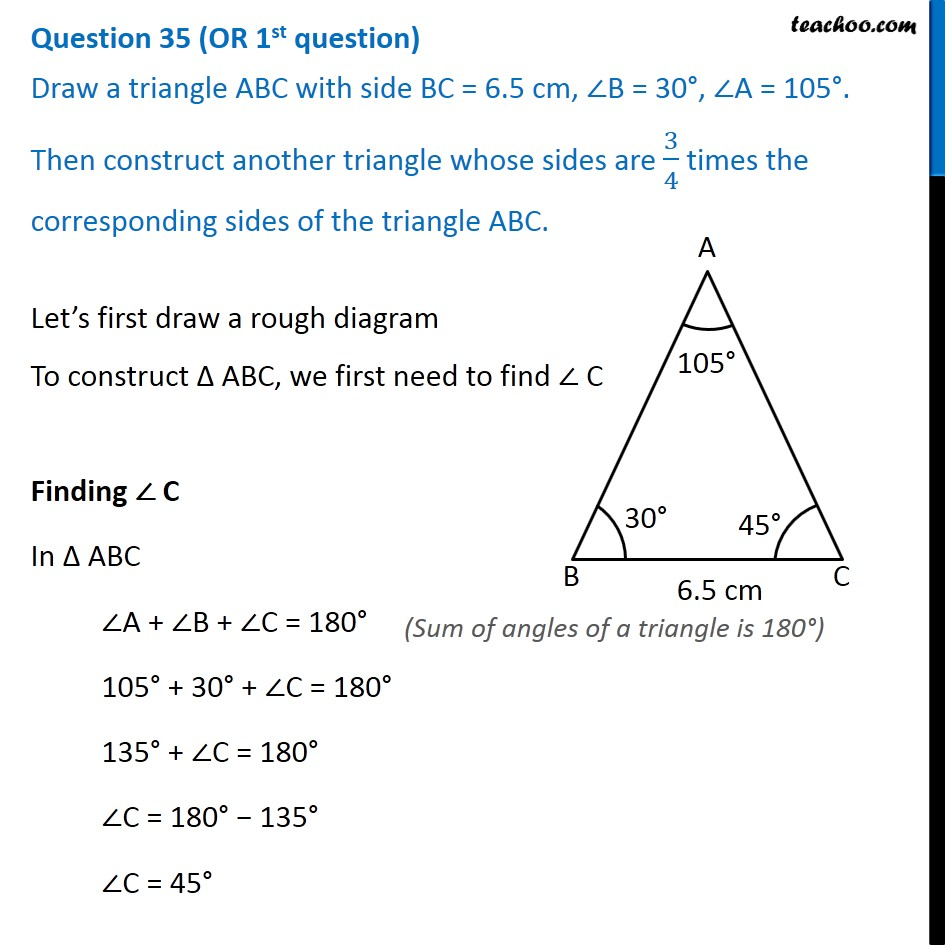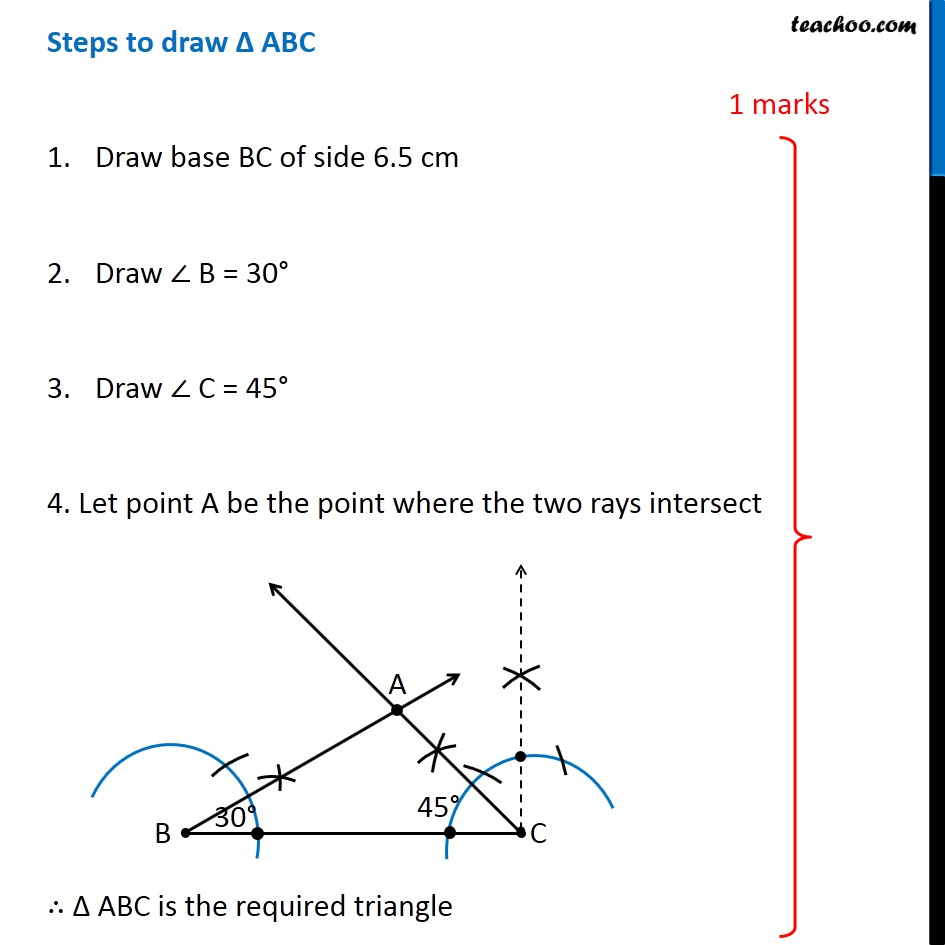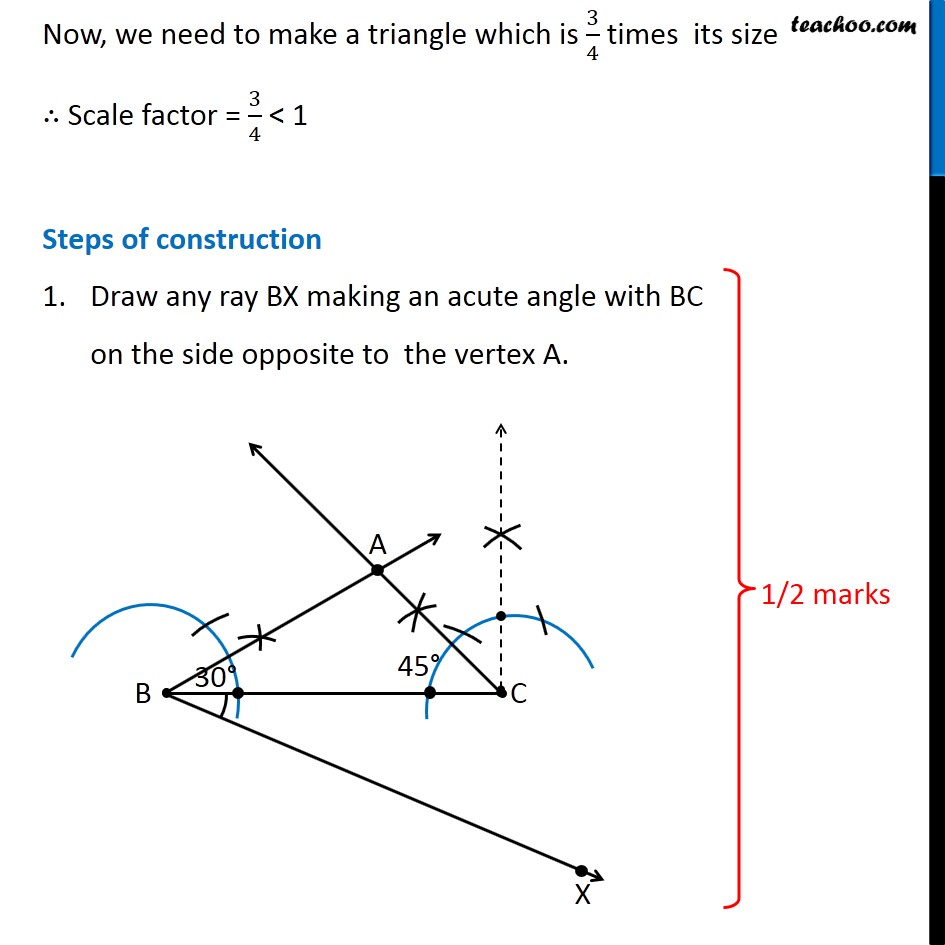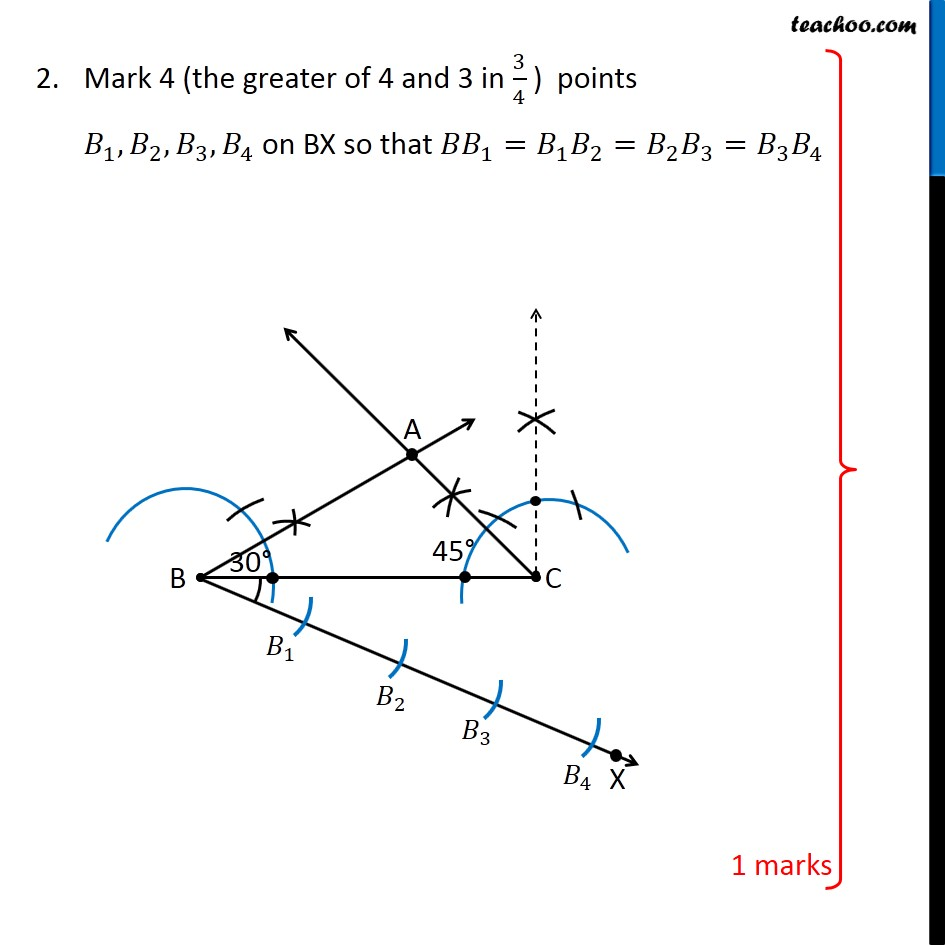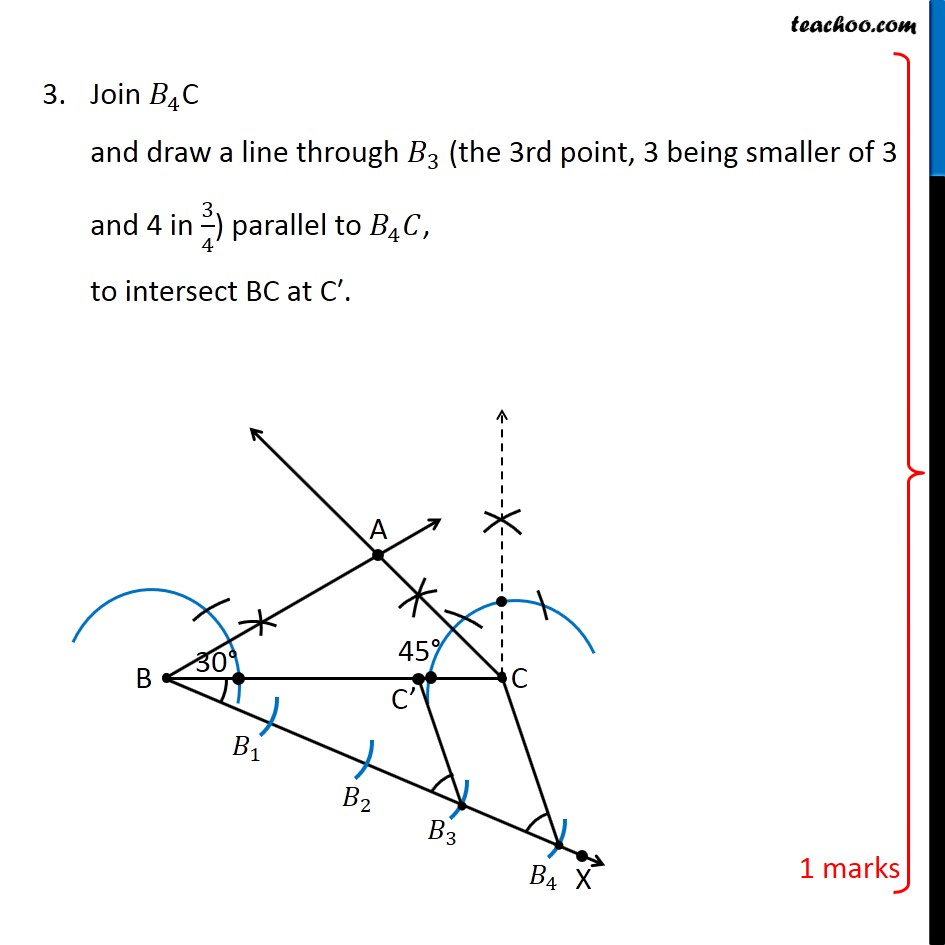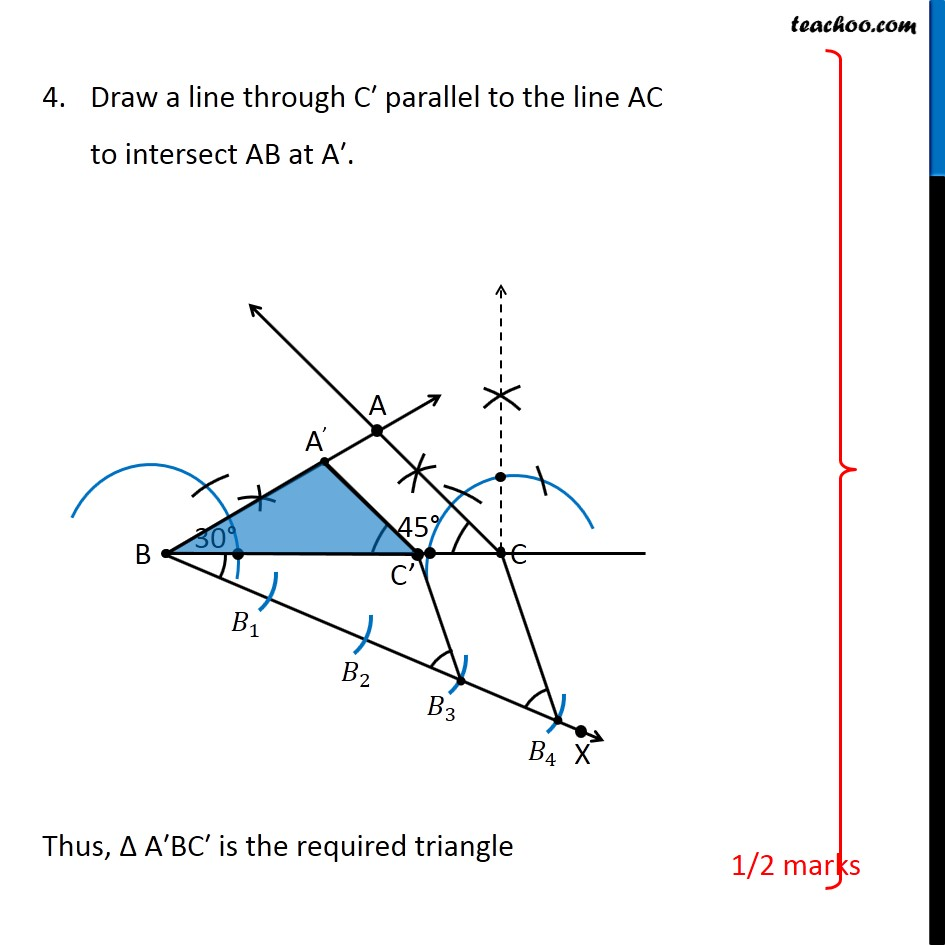Note : This is similar to Ex 11.1, 6 of NCERT – Chapter 11 Class 10

Learn in your speed, with individual attention - Teachoo Maths 1-on-1 Class

### Transcript

Question 35 (OR 1st question) Draw a triangle ABC with side BC = 6.5 cm, ∠B = 30°, ∠A = 105°. Then construct another triangle whose sides are 3/4 times the corresponding sides of the triangle ABC. Let’s first draw a rough diagram To construct Δ ABC, we first need to find ∠ C Finding ∠ C In Δ ABC ∠A + ∠B + ∠C = 180° 105° + 30° + ∠C = 180° 135° + ∠C = 180° ∠C = 180° − 135° ∠C = 45° Steps to draw Δ ABC Draw base BC of side 6.5 cm Draw ∠ B = 30° Draw ∠ C = 45° 4. Let point A be the point where the two rays intersect ∴ Δ ABC is the required triangle Now, we need to make a triangle which is 3/4 times its size ∴ Scale factor = 3/4 < 1 Steps of construction Draw any ray BX making an acute angle with BC on the side opposite to the vertex A. Mark 4 (the greater of 4 and 3 in 3/4 ) points 𝐵_1, 𝐵_2, 𝐵_3,𝐵_4 on BX so that 〖𝐵𝐵〗_1=𝐵_1 𝐵_2=𝐵_2 𝐵_3=𝐵_3 𝐵_4 Join 𝐵_4C and draw a line through 𝐵_3 (the 3rd point, 3 being smaller of 3 and 4 in 3/4) parallel to 𝐵_4 𝐶, to intersect BC at C′. Draw a line through C′ parallel to the line AC to intersect AB at A′. Thus, Δ A′BC′ is the required triangle